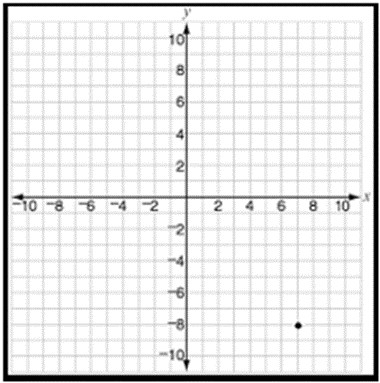# Plotting a point in the coordinate plane

To graph, or plot, points we use two perpendicular lines called the x axis and the y axes.

Horizontal number line is the x axis and the vertical line is the y axis. The part of x axis towards right of origin is the positive x axis and the one towards left of origin is the negative x axis. Similarly the part of y axis above the origin, is the positive y axis and the part of y axis below the origin, is the negative y axis. Every point in the coordinate plane is represented by an ordered pair of x and y coordinates.

Consider the example

Using the pencil, plot the point (−3, 2)

### Solution

The first coordinates tell about the right/left movement from the origin

The second coordinate tells about the up/down movement from the origin.

Since x coordinate is a −3, there is a movement towards the left 3 units from the origin. The y coordinate is 2, which means movement two units vertically up to get to the point (−3, 2).

### Rules to plot a point in the coordinate plane given its ordered pair

• We move along the x axis that many units from the origin left/right as the x coordinates.

• Then we move up/down vertically as many units as the y coordinate to locate the point in the coordinate plane.

Plot the following point in the coordinate plane.

(−9, 5)

### Solution

Step 1 − The x coordinate and y coordinate of the point are −9 and 5 respectively and the point lies in the quadrant 2.

We move 9 units to the left from the origin and then 5 units vertically up to plot the point (−9, 5).

Step 2 − The given point (−9, 5) is plotted in the coordinate plane as follows.Plot the following point in the coordinate plane

(7, −8)

### Solution

Step 1 − The x coordinate and y coordinate of the point are 7 and −8 respectively and the point lies in the quadrant 4.

Step 2 − We move 7 units to the right from origin and then move 8 units vertically down to plot the point (7, −8).

Step 3 − The given point (7, −8) is plotted in the coordinate plane as follows.